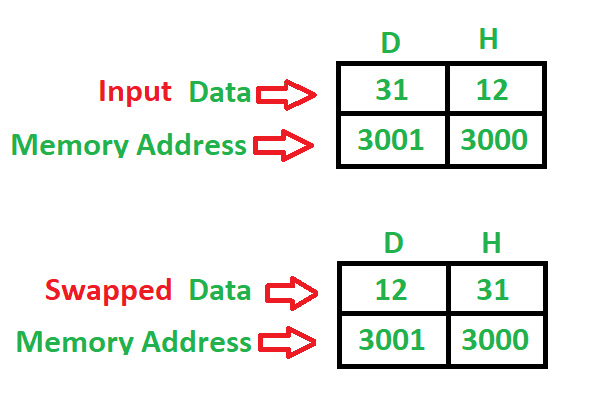# 8085 program to swap two 8 bit numbers using Direct addressing mode

Problem – Write a program to swap two 8-bit numbers using direct addressing mode where starting address is 2000 and the first 8-bit number is stored at 3000 and the second 8-bit number is stored at 3001 memory address. Example –Algorithm –

1. Load a 8-bit number from memory 3000 into accumulator
2. Move value of accumulator into register H
3. Load a 8-bit number from memory 3001 into accumulator
4. Move value of accumulator into register D
5. Exchange both the register pairs
6. Stop

Program –

Explanation – Registers A, H, D are used for general purpose.

1. LDA is used to load accumulator direct using 16-bit address (3 Byte instruction)
2. MOV is used to transfer the data (1 Byte instruction)
3. XCHG is used to exchange the data of both the register pair (H-L), (D-E) (1 Byte instruction)
4. HLT is used to halt the program.

• The program is a useful utility program for swapping two 8-bit numbers using direct addressing mode.

• It is relatively simple and easy to understand, making it useful for teaching purposes.

• It requires fewer instructions than the 16-bit swap program, making it faster and more efficient.